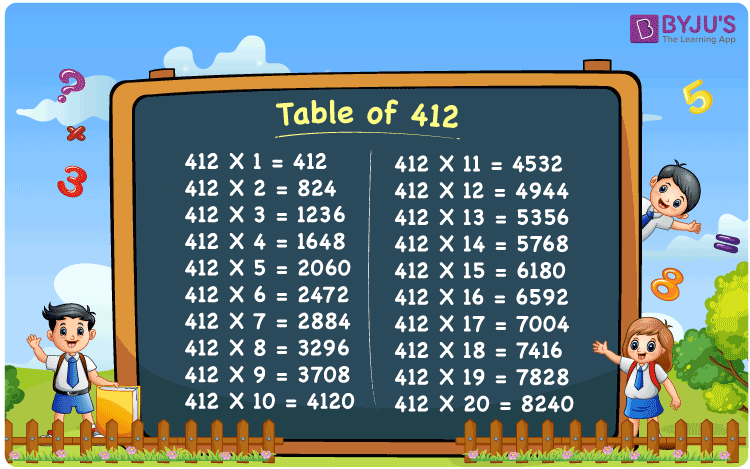Checkout JEE MAINS 2022 Question Paper Analysis : Checkout JEE MAINS 2022 Question Paper Analysis :

# Table of 412

The table of 412 is a multiplication table formed by multiplying 412 by natural numbers. Learning 412 times is important since it facilitates the completion of mathematical tasks quickly. We have included a 412 times chart as well as a tabular version of the 412 multiplication table on this page. You can download the PDF for the table of 412 by clicking the link below.

## Table of 412 Chart## What is the 412 Times Table?

The 412 times table is also known as the table of 412, which is obtained by repeatedly adding 412 for a certain number of times.

For example, 412 × 3 = 1236.

It can also be written as 412 + 412 + 412 = 1236.

Thus, the below table shows the 412 times table in the repeated addition form.

 412×1 = 412 412 412×2 = 824 412 + 412 = 824 412×3 = 1236 412 + 412 + 412 = 1236 412×4 = 1648 412 + 412 + 412 + 412 = 1648 412×5 = 2060 412 + 412 + 412 + 412 + 412 = 2060 412×6 = 2472 412 + 412 + 412 + 412 + 412 + 412 = 2472 412×7 = 2884 412 + 412 + 412 + 412 + 412 + 412 + 412 = 2884 412×8 = 3296 412 + 412 + 412 + 412 + 412 + 412 + 412 + 412 = 3296 412×9 = 3708 412 + 412 + 412 + 412 + 412 + 412 + 412 + 412 + 412 = 3708 412×10 = 4120 412 + 412 + 412 + 412 + 412 + 412 + 412 + 412 + 412 + 412 = 4120

## Multiplication Table of 412

The multiplication table of 412 is provided here up to 20 times in the tabular form..

 412 × 1 = 412 412 × 2 = 824 412 × 3 = 1236 412 × 4 = 1648 412 × 5 = 2060 412 × 6 = 2472 412 × 7 = 2884 412 × 8 = 3296 412 × 9 = 3708 412 × 10 = 4120 412 × 11 = 4532 412 × 12 = 4944 412 × 13 = 5356 412 × 14 = 5768 412 × 15 = 6180 412 × 16 = 6592 412 × 17 = 7004 412 × 18 = 7416 412 × 19 = 7828 412 × 20 = 8240

## Solved Example on the Table of 412

Example:

Determine the value of c, if 412c = 5768.

Solution:

Given that, 412c = 5768.

Hence, c = 5768/412

c = 14

Therefore, the value of c is 14.

## Frequently Asked Questions on the Table of 412

### What is the table of 412?

The table of 412 is the multiplication table, which displays the result as the multiples of 412.

### Write the 412 times table.

412×1 = 412, 412×2 = 824, 412×3 = 1236, 412×4 = 1648, 412×5 = 2060, 412×6 = 2472, 412×7 = 2884, 412×8 = 3296, 412×9 = 3708, 412×10 = 4120.

### What is 412 times 19?

412 times 19 is 7828. I.e., 412 × 19 = 7828.